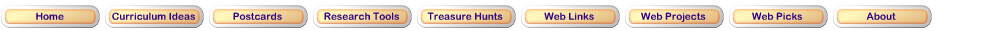Number and Operations Standard

Understand meanings of operations and how they relate to one another
• understand various meanings of addition and subtraction of whole numbers and the relationship between the two operations;
• understand the effects of adding and subtracting whole numbers;
• understand situations that entail multiplication and division, such as equal groupings of objects and sharing equally.

Visit these Websites to practice addition and subtraction.AAA Math Practice your skills using one of the addition or subtraction activities.Math Monsters Practice addition with this game.Math Monsters Practice subtraction with this game..Marble Math Addition with manipulatives.Math Magician Games Take the math challenge.Updated March 14, 2017
© 1996 - 2015 Linda C. Joseph